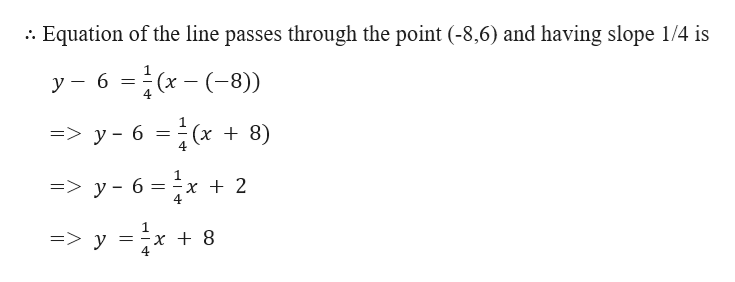# The slope is 1/4 and the line passes through the point (-8,6). Give your answer in slope-intercept form.

Question
33 views

The slope is 1/4 and the line passes through the point (-8,6). Give your answer in slope-intercept form.

check_circle

Step 1

Given,

The slope is 1/4 and the line passes through the point (-8,6).

We know that the equation of the...help_outlineImage TranscriptioncloseEquation of the line passes through the point (-8,6) and having slope 1/4 is - 6-(x- (-8)) 4 1 =>y- 6 (x8) 4 => y-6 x +2 x + 8 => y = fullscreen

### Want to see the full answer?

See Solution

#### Want to see this answer and more?

Solutions are written by subject experts who are available 24/7. Questions are typically answered within 1 hour.*

See Solution
*Response times may vary by subject and question.
Tagged in

### Equations and In-equations